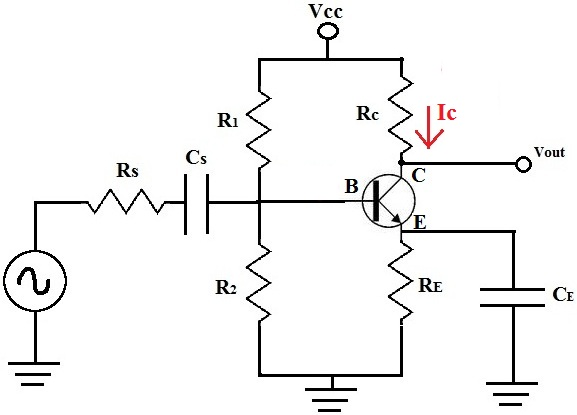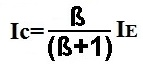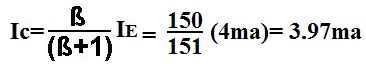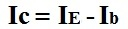﻿ How to Calculate the Collector Current, Ic, of a Transistor# How to Calculate the Collector Current, Ic, of a TransistorThe collector current, Ic, of a transistor is the amplified output current of a bipolar junction transistor.

There are several ways to find the collector current, Ic, of a transistor. And it all depends on what information is already known about the transistor:

### 1st Way to Calculate Collector Current Ic

Using Known Values

If the base current, Ib, and β are known, then Ic can be computed by the following formula:Example
If Ib=35μa and β=100, then Ic calculates out to be:### 2nd Way to Calculate Collector Current Ic

Using Known Values

If the emitter current, Ie, and β are known, then Ic can be computed by the following formula:Example
If Ie=4ma and β=150, then the value of Ic is computed to be:### 3rd Way to Calculate Collector Current Ic

Using Known Values
If base current, Ib, and emitter current, Ie, are known, then Ic can be calculated by the formula:Example
If Ie=4ma and Ib=30μa, then Ic calculates out to be:Related Resources